# Scanner - Utility Analysis (Theory of Consumer Behaviour) Notes | Study Economics Class 11 - Commerce

## Commerce: Scanner - Utility Analysis (Theory of Consumer Behaviour) Notes | Study Economics Class 11 - Commerce

The document Scanner - Utility Analysis (Theory of Consumer Behaviour) Notes | Study Economics Class 11 - Commerce is a part of the Commerce Course Economics Class 11.
All you need of Commerce at this link: Commerce

N.C.E.R.T

(Q1) Define Total Utility and marginal Utility ?

(Q2) What is Consumer Equilibrium and state its Condition ?

(Q3) Starting from an initial situation of consumer’s equilibrium, suppose that the marginal utility of a rupee increases . Will it increase or decrease the quantity demanded of the product ?

(Q4) Ice Cream sells for Rs 30. Lakshmi, who loves ice cream, has already eaten 3. Her marginal utility from eating 3 ice cream is 90. Suppose further that for her marginal utility of one rupee is 3. Should she eat more ice cream or should she stop ?

(Q5) Ice cream sells for Rs 20. Mohini who likes ice cream, has already consumed 4. Her marginal utility of one rupee is 4. Should she consume more ice cream or stop the consumption

(Q6) Explain why consumer’s equilibrium is attained when the marginal utility of a product in terms of money is equal to its price

(Q7) TU is maximum when MU is zero ? Explain

(Q8) How is equilibrium of the consumer affected when MUM happens to rise, and PX is constant?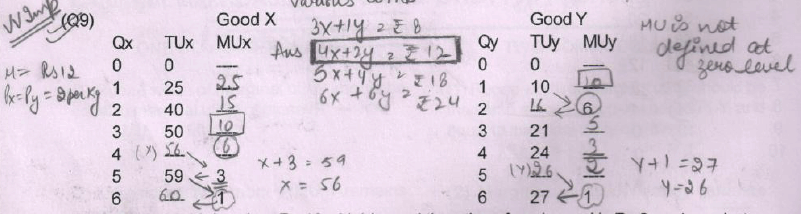Suppose that Mr Mohan has Rs 12 with him and the price of each good is Rs 2 per kg , what
is the utility maximising combination of X and Y goods ?.

C.B.S.E Questions

(Q1) How does a consumer reach equilibrium position when he is buying only one commodity ?
Explain with the help of marginal utility schedule ?

(Q2) What is meant by Consumer Equilibrium ? State its condition in case of single commodity

(Q3) Define Marginal Utility ? State the law of diminishing marginal utility ?

Sample Paper & C.B.S.E 2010 Questions

(Q1) Given below is the utility schedule of a consumer for commodity X.The price of the commodity is Rs. 6 per unit. How many units should the consumer purchase to maximize satisfaction?
(Assume that utility is expressed in utils and 1 util = Re. 1). Give reasons for your answer.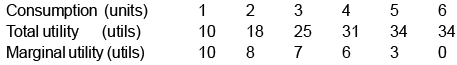(Q2) What is consumer’s equilbrium? Explain the conditions of consumer.s equilibrium assuming
that the consumer consumes only two goods.

(Q3) Given the market price of a good, how does a consumer decide as to how many units of

C.B.S.E 2011 & 2012 Questions

(Q1) Explain the law of diminishing marginal utility with the help of a total utility schedule.(3M)

(Q2) A consumer consumes only two goods X and Y. At a consumption level of these two goods,he finds that the ratio of marginal utility to price in case of X is higher than in case of Y. Explain the reaction of the consumer.(4M)

(Q3) Explain the conditions determining how many units of a good the consumer will buy at a given price.(4M)

(Q4) A consumer consumes only two goods X and Y and is in equilibrium. Price of X falls.Explain the reaction of the consumer through the Utility Analysis.(3 M)

(Q5) A consumer consumes only two goods X and Y and is in equilibrium . Price of X rises.Explain the reaction of the consumer through the utility analysis .CBSE (F) 2012 (4M)

(Q6) State true or false “ Marginal utility can never be negative”

Sample Paper 2014-15

(Q1) What does the area under the marginal utility curve depict ?

(a)  Average Utility      (b) Total Utility  (c)  Indifference Curve   (d)  Consumer equilibrium

Ans: (c)

(Q2) Which of the can be referred to as ‘point of satiety’?

(i)  Marginal Utility is negative (ii) Marginal utility is zero (iii) Total Utility is rising (iv) Total Utility is falling

Ans: (ii) Marginal Utility is zero

(Q3) When is a consumer said to be rational? (1)

Ans :: A consumer is said to be ‘rational’ when he aims at maximizing his utility from the consumption of the given commodity, within his money income

(Q4) A  consumer consumes two goods X and Y. What will happen if MUx/Px is greater than MUy/Py ? (4)

Ans: If MUx/Px is greater than MUy/Py, then it means that the satisfaction a consumer derives from spending a rupee on Good x is greater than the satisfaction derived from spending a rupee on Good Y. (1)

The consumer will relocate her income – substitute Good X for Good Y. As the consumption of Good X increases its marginal utility will fall. As the consumptionof Good Y decreases, it marginal utility will increase. This is due to the law of diminishing marginal utility.(1)

This process will continue till MUx/Px becomes equal to MUy/Py and the consumer is inequilibrium. (1)

(Q5) A consumer, Mr. Aman is in state of equilibrium consuming two goods X and Y, with given prices Px and Py. Explain what will happen if :

(a) MUx / Px is greater than MUy / Py    (b) Py falls

Ans: (a)

(b)  If Py falls, MUx/Px < MUy/Py, then it means that satisfaction derived from spendinga rupee on Good X is lesser than the satisfaction derived from spending a rupee on Good Y.
Mr. Aman will reallocate his income by substituting Good Y for Good X.
As the consumption of Good Y increases the marginal utility derived from it goes on diminishing and reverse proposition occurs for Good X, this process will continue till MUx/Px becomes equal to MUy/Py.

C.B.S.E & Sample Paper 2013

(Q1) Explain the conditions of consumer’s equilibrium under utility analysis.(3 M)

(Q2) A consumer consumes only two goods X and Y and is in equilibrium. Price of good Y falls.Show that it will lead to rise in demand for good Y(C)

C.B.S.E Paper 2014

(Q1) A consumer consumes only two goods X and Y and is in equilibrium. Show that when the price of good X rises, the consumer buys less of good X. Use utility analysis.

Ans: According to the utility analysis, the consumer is in equilibrium when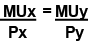Now, given that Px rises, thenSince per rupee MUx is lower than per rupee MUy  , the consumer will buy less of X and more of Y. →It shows that when Px rises, demand for X falls.

(Q2) Given the price of a good, how will a consumer decide as to how much quantity of that good to buy ? Use utility analysis. (6M)

Ans: W hile buying a unit of a good the consumer compares price with marginal utility (MU).
→So long as the price the consumer is willing to pay (i.e. MU) is higher than the marketprice, the consumer will go on buying. As the consumer buys more, MU falls due to the law of Diminishing Marginal Utility.

→ The consumer stops buying when MU or Price he is willing to pay is equal to marketprice i.e. MU = P.

→  If the consumer still buys more, MU will become lower than price; a position a rationalconsumer will not accept.

(Q3) A consumer consumes only two goods. Explain the conditions of consumer’s equilibrium with the help of the Utility Analysis. (4M)

Ans: Assuming that the only two goods the consumer consumes are X and Y, the conditions of equilibrium are :

(1) Mux / Muy = Px /Py (1)

(2) MU falls as more is consumed     (1/2)

Explanation : (1) Suppose .Mux / Muy > Px /Py       he consumer will not be in equilibrium because per rupee MU of X is greater than per rupee MU of Y. This will induce the consumer to buy more of X by reducing expenditure on Y. It will lead to fall in MUx and rise in MUy. This will continue till Mux / Muy =Px /Py (2)

(2) Unless MU falls as more of a good is consumed the consumer will not reach equilibrium. (1/2)
(Explanation based on Mux / Muy < Px /Py is also correct.)

Sample Paper 2015

(Q1) What does the area under the marginal utility curve depict ?
(a) Average Utility (b) Total Utility
(c) Indifference Curve (d) Consumer equilibrium

Ans:  (c)

(Q2) Which of the can be referred to as ‘point of satiety’?

(i)  Marginal Utility is negative (ii) Marginal utility is zero (iii) Total Utility is rising (iv) Total Utility is falling

Ans: (ii) Marginal Utility is zero

(Q3) When is a consumer said to be rational? (1)

Ans: A consumer is said to be ‘rational’ when he aims at maximizing his utility from the consumption of the given commodity, within his money income

(Q4) A  consumer consumes two goods X and Y. What will happen if MUx/Px is greater than MUy/Py ? (4)

Ans: If MUx/Px is greater than MUy/Py, then it means that the satisfaction a consumer derives from spending a rupee on Good x is greater than the satisfaction derived from spending a rupee on Good Y. (1)

(Q2) A consumer consumes two goods X and Y. What will happen if MUx/Px is greater than MUy /Py ?( 4M)

C.B.S.E Paper 2015

(Q1) A consumer consumes only two goods X and Y whose prices are Rs. 5 and Rs. 4 respectively. If the consumer chooses a combination of the two goods with marginal utility of X equal to 4 and that of Y equal to 5, is the consumer in equilibrium ? Why or why not ? What will a rational consumer do in this situation ? Use utility analysis.

Ans: Given Px = 5 , Py = 4 and MUx = 4 , MUy = 5 , the consumer will be in equilibrium when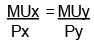Substituting values, we find that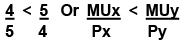The consumer is not in equilibrium.

Since per rupee MUx is lower than per rupee MUy , the consumer will buy less of x and more of y. As a result due to Law of Diminishing Marginal Utility, MUx will rise and MUy will fall till(Diagram not required)

(Q2) A consumer consumes only two goods X and Y whose prices are Rs.2 and Rs.1 per unit respectively. If the consumer chooses a combination of the two goods with marginal utility of X being 4 and that of Y also being 4, is the consumer in equilibrium? Give reasons. Explain whatwill a rational consumer do in this situation. Use marginal utility analysis.(F)

(Q3) What is ‘ordinal’ utility?

(Q4) Which of the can be referred to as ‘point of satiety’? [SP (1M)]

(i) Marginal Utility is negative (iii) Total Utility is rising
(ii) Marginal utility is zero (iv) Total Utility is falling

(Q5) A consumer consumes one good and is in equilibrium. Show that when price of that good falls, he buys more of that good. Use utility approach.[Delhi (C)]

CBSE 2016

(Q1) A consumer consumes only two goods X and Y. Explain the conditions of consumer’s equilibrium using Marginal Utility Analysis.

(Q2) Define utility. Explain the Law of Diminishing Marginal Utility. (4M)

Ans: Utility refers to the amount of satisfaction derived from consumption of a good     (1)

As the consumer goes on consuming more and more units of a good , utility obtained from consuming each extra unit goes on falling. Ultimately , it may become zero or even negative . This is the law of diminishing marginal utility    (3)

(Q3) A consumer consumes only two goods X and Y. Marginal utilities of X and Y are 3 and 4 respectively. Prices of X and Y are Rs.4 per unit each. Is consumer in equilibrium ? What will be further reaction of the consumer ? Give reasons.

(Q4) A consumer consumes only two goods X and Y. Marginal utilities of X and Y are 4 and 3 respectively. Price of X and Price of Y is Rs.3 per unit. Is consumer in equilibrium ? What will be further reaction of the consumer ? Give reasons. (3M)

(Q5) A consumer consumes only two goods X and Y. The Marginal utilities of X and Y is 3.Prices of X and Y are Rs.2 and Rs.1 respectively. Is consumer in equilibrium ? What will be further reaction of the consumer ? Give reasons. (3M)

(Q6) A consumer consumes only two goods X and Y. Marginal utilities of X and Y are 5 and 4 respectively. The prices of X and Y are Rs. 4 per unit and Rs. 5 per unit respectively. Is the consumer in equilibrium? What will be the further reaction of the consumer ? Explain.  (3)

N.C.E.R.T Questions

(Q1) What do you mean by the budget set of a consumer ?

(Q2) What is budget line?

(Q3) Explain why the budget line is downward sloping.

(Q4) A consumer wants to consume two goods. The prices of the two goods are Rs. 4 and Rs. 5 respectively. The consumer’s income is Rs. 20.

(i) Write down the equcation of the budget line.

(ii) How much of good 1 can the consumer consume if she spends her entire income on that good?

(iii) How much of good 2 can she consume if she spends her entire income on that good?

(iv) What is the slope of the budget line?

(Q5) How does the budget line change if the consumer’s income increases to Rs 40 but the prices remain unchanged?

(Q6) How does the budget line change if the price of good 2 decreases by a rupee but the price of good 1 and the consumer’s income remain unchanged?

(Q7) What happens to the budget set if both the prices as well as the income double ?

(Q8) Suppose a consumer can afford to buy 6 units of good 1 and 8 units of good 2 if she spends her entire income. The prices of the two goods are Rs 6 and Rs 8 respectively. How much is the consumer’s income ?

(Q9) Show diagrammatically consumer’s equilibrium using indifference curve analysis.

(Q10) What do you mean by ‘monotonic preferences’?

(Q11) If a consumer has monotonic preferences, can she be indifferent between the bundles (10, 8) and (8, 6)?

(Q12) Suppose your friend is indifferent to the bundles (5, 6) and (6, 6). Are the preferences of your friend monotonic?

(Q13) A consumer spends his entire income on food and clothing. Draw the budget line for each of the following situations, identifying the intercepts and slopes in each case (measure food consumption on horizontal axis).

(a) Monthly income is Rs. 5,000. The price of food is Rs. 100 and of clothing is Rs. 250.

(b) Same condition as in part (a), except that income is Rs. 4,000.

(Q14) Navin’s budget line relating to good X and good Y has intercepts of 150 units of X and 120 units of Y. If the price of good X is Rs. 12,

(a) What is Navin’s money income ?

(b) What is the price of good Y?

(c) What is the slope of the budget line?

(Q15) Explain consumer’s equilibrium with the help of Indifference Curves. Vandana spends her entire monthly income of Rs. 600 on two commodities X and Y. The price of X is Rs. 30 and price of Y is Rs. 10. If the consumes 12 units of X and 24 units of Y, her MRS is (1Y/1X). Is she in equilibrium at this point on her budget line ?

(Q16) Suppose that the price of a commodity Y is Re.1 per unit and the price of commodity X is Rs.2 per unit. Suppose further that an individual’s money income is Rs. 16 per time period and all is spent on X and Y.

(i) Draw the Budget Line for the consumer.

(ii) Find the slope of the Budget Line.

(iii) Write the equation of the Budget Line.

(Q17) Sanjiv’s budget line relating to good X and good Y has intercepts of 40 units of good X and 20 units of good Y. If the price of the good X is Rs. 8; what is Sanjiv’s income ? Calculate the price of good X and slope of the budget line.

(Q18) Distinguish between attainable (or feasible) and non-attainable (or non-feasible) combinations of a set of two goods. Draw a suitable diagram.

CBSE 2010 + Sample Paper Questions

(Q1) Define a budget line. (1M)

(Q2) Define an indifference curve. (1M)

(Q3) Define an indifference map. (1M)

(Q4) What are the conditions of consumer’s equilibrium under the indifference curve approach ? What changes will take place if the conditions are not fulfilled to reach equilibrium ? (6M)

(Q5) (a) What is a budget line? What does the point on it indicate in terms of prices?

(b) A consumer consumes only two goods X and Y. Her money income is Rs 24 and the prices of Goods X and Y are Rs 4 and Rs 2 respectively. Answer the following questions:

(i) Can the consumer afford a bundle 4X and 5Y ? Explain

(ii) W hat will be the MRSXY when the consumer is in equilibrium?  Explain. (6 M)

CBSE 2011 & CBSE 2012

(Q1) Define a budget line. (1 mark)

(Q2) Define a budget line. When can it shift to the right ? (4 marks)

(Q3) What is budget set ? Explain what can lead to change in budget set. (4 marks)

(Q4) Explain the concepts of (i) marginal rate of substitution and (ii) budget line equation with the help of numerical examples. (6 marks)

(Q5) Explain any three properties of Indifference Curves. (6 marks)

(Q6) Explain the conditions of consumer’s equilibrium with the help of the Indifference Curve Analysis.(6 marks)

(Q7) Explain the concept of Marginal Rate of substitution . Explain the reaction of the consumer when Marginal Rate of substitution is higher than the ratio of prices (6M)

(Q8) Explain the difference between cardinal utility and ordinal utility. Give example in each case.(6 marks)

(Q9) Explain the concept of Marginal Rate of substitution by giving an example . What happens to MRS when consumer moves downwards along the indifference curve ? Give reasons for your answer. CBSE (D) 2011

(Q10) What are Monotonic preferences ? Explain why is an indifference curve (i) Slopes downward from left to right (ii) Convex CBSE (D) 2011

(Q11) What are monotonic preferences ? Explain why an indifference curve to the right shows higher utility.(F)

(Q12) State true or false    (a) A budget set is the collection of all bundles of goods that  a consumer wants to buy   (b) A budget set is the collection of such bundles of goods that  give same satisfaction

SAMPLE  PAPER 2014-15  & 15-16 & 16-17

(Q1) Explain why an indifference curve is convex to the origin? (4)

Ans :: An indifference curve is convex to the origin due to diminishing MRS .  Diminishing MRS means that the number of units of ‘Good Y’ that a consumer wants tosubstitute for one extra unit of ‘Good X’ goes on decreasing as the consumption of Good X increases.  As consumption of Good X increases, the willingness to pay for it diminishes (due to the lawof diminishing marginal utility). This payment is in terms of the units of Good Y sacrificed. Thus, MRS diminishes along an indifference curve, which makes it convex to the origin.

(Q2) A consumer consumes only two goods. Explain the conditions that need to be satisfied for the consumer to be in equilibrium under indifference curve analysis. (6)

(Q3) ‘Higher indifference curve represents higher level of satisfaction to the consumer’. Explain the statement, also state the underlying assumption related to this property of indifference curve.

(Q4) Show diagrammatically the conditions for consumer’s equilibrium, in Hicksian analysis of demand.(6M)

(Q5) Identify which of the following is not true for the Indifference Curves. Give valid reasons for choice of your answer: a) Lower indifference curve represents lower level of satisfaction. b) Two regular convex to origin indifference curves can intersect each other. c) Indifference curve must be convex to origin at the point of tangency with the budget line at the consumer’s equilibrium. d) Indifference curves are drawn under the ordinal approach to consumer equilibrium.

(Q6) A consumer has total money income of 250 to be spent on two goods X and Y with prices of 25 and 10 per unit respectively. On the basis of the information given, answer the following questions: (a) Give the equation of the budget line for the consumer. (b) What is the value of slope of the budget line ? (c) How many units can the consumer buy if he is to spend all his money income on good X ? (d) How does the budget line change if there is a fall in price of good Y ? (4)

C.B.S.E 2013

(Q1) Explain the meaning of diminishing marginal rate of substitution with the help of a numerical example. (3 M)

(Q2) Explain three properties of indifference curves.

(Q3) Explain the conditions of consumer’s equilibrium under indifference curve approach. (6 M)

(Q4) Why is an indifference curve convex towards the origin ? Explain.         (3 marks)

(Q5) A consumer consumes only two goods. For the consumer to be in equilibrium why must Marginal Rate of Substitution between the two goods must be equal to the ratio of prices of these two goods ? Is it enough to ensure equilibrium ? (6 M)

(Q6) A consumer consumes only two goods. Why is the consumer said to be in equilibrium when he buys only that combination of the two goods which lies at that point on the Indifference curve where the budget line is tangent to the indifference curve ? Explain. Use diagram (6 M)

C.B.S.E PAPER 2014 & CBSE 2015

(Q1) Define indifference curve. (1M)

Ans: It is the locus of points that represent different combination of two goods that give the same satisfaction to the consumer.

(Q2) Define Budget set

Ans: Budget set consists of all the bundles of the goods which at given prices cost less than or equal to the given income of the consumer.

(Q3) What is meant by monotonic preferences. (1M)

Ans: Monotonic preferences refers to a situation when consumption increases total utility also increases alongwith.

(Q4) Define Budget line Ans :: Budget line is the locus of points, each point representing a bundle of the two goods which cost the consumer exactly his income.

(Q5) Define Indifference map

Ans: The set of indifference curves of a consumer is called indifference map.

(Q6) Explain why is an indifference curve (a) downward sloping and (b) convex.

(Q7) Explain the concept of ‘Marginal Rate of Substitution’ with the help of a numerical example.Also explain its behaviour along an indifference curve. (6M)

Ans: Marginal Rate of Substitution (MRS) means the rate at which a consumer is willing to sacrifice quantity of one good to obtain one more unit of the other good. Let the two goods consumed be A and B. (1M) Suppose the following combinations of these two goods have the same utility level for him : (3M)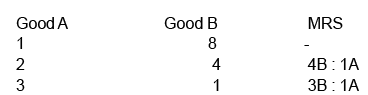The consumer is willing to sacrifice 4B to obtain second unit of A. For the third unit of A. he is willing to sacrifice less because marginal utility of A decreases as he consumes more of A. (2M)

(Q8) State the conditions of consumer’s equilibrium in the Indifference Curve Analysis and explain the rationale behind these conditions. (6M)  (F)

(Q9) Explain the distinction between equations of budget line and budget constraint.  (c)

(Q10) A consumer consumes only two goods . If the price of the goods falls , the indifference curve (a) Shift upwards (b) Shifts downards (c) Can shift both upwards or downwards (d) does not shift

(Q11) If marginal rate of substitution is constant throughout, the indifference curve will be : (choose the correct alternative) (D) (a) parallel to the X-axis (b) downward sloping concave (c) downward sloping convex (d) downward sloping straight line

Ans: (d) Downward sloping straight line.

(Q12) A consumer consumes only two goods. If price of one of the goods falls, the indifference curve: (choose the correct alternative) (F) (a) shifts upwards (b) shifts downwards (c) can shift both upwards or downwards (d) does not shift

Ans: (d)

(Q13) If Marginal Rate of Substitution is increasing throughout, the Indifference Curve will be : (Choose the correct alternative) (1 M) (a) Downward slopping convex (b) Downward sloping concave (c) Downward sloping straight line (d) Upward sloping convex

Ans: (b) Downward sloping concave.

(Q14) Give equation of budget line. (D) Ans :: p1x1 + p2x2 = m

(Q15) Give equation of budget set. (D) Ans :: p1x1 + p2x2 < m

(Q16) Define utility Ans :: Utility refers to satisfaction from the consumption of goods.

(Q17) What do you mean by ordinal utility Ans :: Ordinal utility is the utility expressed in ranks.

(Q18) What are monotonic preferences ?

Ans: Monotonic preferences mean that as consumption increases total utility also increases alongwith.

(Q19) Define indifference curve. (1M) Ans :: It is the locus of points representing such bundles of two goods, among which the consumer is indifferent.

(Q20) A consumer consumes only two goods X and Y, both priced at Rs. 2 per unit. If the consumer chooses a combination of the two goods with Marginal Rate of Substitution equal to 2, is the consumer in equilibrium ? Why or why not ? What will a rational consumer do in this situation ? Explain.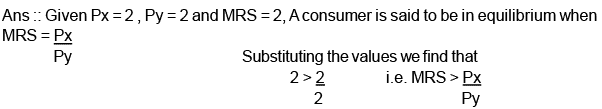MRS > Px / Py means that consumer is willing to pay more for one more unit of X as compared to what the market demands. The consumer will buy more and more of X. As a result MRS will fall due to the Law of Diminishing Marginal Utility. This will continue till MRS = Px / Py and consumer is in equilibrium. (3M)

(Q21) A consumer consumes only two goods, each priced at rupee one per unit. If the consumer chooses a combination of the two goods with marginal rate of substitution equal to 2, is the consumer in equilibrium? Give reasons. Explain what will a rational consumer do in this situation.

(Q22) A consumer consumes only two goods X and Y both priced at Rs.3 per unit. If the consumer chooses a combination of these two goods with marginal rate of substitution equal to 3, is the consumer in equilibrium ? Give reasons. What will a rational consumer do in this situation? Explain

CBSE 2017

(Q1) A consumer consumes only two goods. Explain the conditions of consumer’s equilibrium using utility analysis.

(Q2) When marginal utility is zero, total utility is

(a) zero
(b) minimum
(c) maximum
(d) negative

CBSE 2018

(Q1) Given the price of a good, how will a consumer decide as to how much quantity to buy of that good ? Explain.

(Q2) A consumer consumes only two goods X and Y. Explain the conditions of consumer’s equilibrium using Utility Analysis.   (6M)

The document Scanner - Utility Analysis (Theory of Consumer Behaviour) Notes | Study Economics Class 11 - Commerce is a part of the Commerce Course Economics Class 11.
All you need of Commerce at this link: CommerceUse Code STAYHOME200 and get INR 200 additional OFF

## Economics Class 11

201 videos|195 docs|64 tests

Track your progress, build streaks, highlight & save important lessons and more!

,

,

,

,

,

,

,

,

,

,

,

,

,

,

,

,

,

,

,

,

,

;